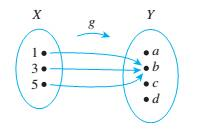Chapter 7.1, Problem 1ES### Discrete Mathematics With Applicat...

5th Edition
EPP + 1 other
ISBN: 9781337694193

#### Solutions

Chapter
Section### Discrete Mathematics With Applicat...

5th Edition
EPP + 1 other
ISBN: 9781337694193
Textbook Problem
1 views

# Let   X   =   { l ,   3 ,   5 } and Y   =   { a ,   b ,   c ,   d ) . Define g   :   X → Y by the following arrow diagram.Write the domain of g and the co-domain of g. Find g(1), g(3), and g(5). What is the range of g? Is 3 an inverse image of a? Is I an inverse image of b? What is the inverse image of b? of c? Represent g as a set of ordered pairs.

To determine

(a)

Write the domain of f and the co-domain of f.

Explanation

Given information:

Concept used:

f(x)=yHere, x:domainy:codomain

Calculation:

The given function f is from the set X={1,3,5

To determine

(b)

Find f(1),f(3) and f(5)

To determine

(c)

What is the range of f

To determine

(d)

Is 3 an inverse image of s ? Is 1 an inverse image of u ?

To determine

(e)

What is the inverse image of s ? Of u ? Of v ?

To determine

(f)

Represent f as a set of ordered pairs.

### Still sussing out bartleby?

Check out a sample textbook solution.

See a sample solution

#### The Solution to Your Study Problems

Bartleby provides explanations to thousands of textbook problems written by our experts, many with advanced degrees!

Get Started